## Monday, March 18, 2013

### Should You Rebalance Your Investment Portfolio?

Investors are often advised to rebalance their portfolios periodically in order to maintain their target asset allocations.
Translated, this means the following:
·         Suppose we want to have 20% of our investments in global equity, 40% in domestic equity, and 40% in fixed income,
·         A year of time goes by,
·         Each of our investment classes has changed in value. Some went up, others went down.
·         Because of this, our last investment statement shows our current portfolio is 25% global equity, 42% domestic equity and 33% fixed income.
·         We sell 5% of our global equity holdings, sell 2% of our domestic equity holdings, and use those proceeds to buy more fixed income assets, so that in the end we are back to a 20%/40%/40% mix of assets.
Undertaking these transactions to get back to our target levels is called rebalancing.
But what happens if we don’t? How much performance difference does the act of rebalancing create versus buying and holding? If we are making periodic contributions to the portfolio, such as funding a pension plan or payroll deductions into a 401k, won’t the fact that we are investing periodically at different prices take care of this and eliminate the need to rebalance?
Monte-Carlo simulation can give us a quantitative perspective to these questions.

Portfolio Math…Briefly
Figure A
Portfolio Returns: Mean and VarianceFigure A shows the equations we will need to use to calculate our portfolio data.
Equation 1 calculates the return to our portfolio. For each of the securities in our portfolio (n securities in total), we multiply the weight of that security by the return for that security and simply add them all together.
Either Equation 2 or 3 calculates the variance of our portfolio. This equation is a little more complicated because the variation between securities can be correlated. If security a goes up every time security b goes up, then our variance will be magnified. If security a goes down every time security b goes up, then our variance will be a lot smaller.
Variance and its companion metric – standard deviation (which is just the square root of variance) - is the measure of our portfolio risk. We have calculated this measure in prior posts (see), but stated in words variance is a measure of how close or far each of the returns are from the average.
For example, look at the following 2 data sets:
A          {4,5,6}
B          {0,5,10}
Each data set has an average of 5. A’s first element is 4, which is 1 away from 5. B’s first element is 0, which is 5 away from 5. Thus, B will have a much higher variance and standard deviation because its data elements are “farther away” from the average than A’s.

The Efficient Frontier
A portfolio can be constructed in an almost infinite number of combinations, however very few are “best in their class”.
Figure B
Portfolio Efficient FrontierFigure B shows a graph with Return on the y-axis and Risk on the x-axis. Each of the three letters A, B, and C represents three different portfolio combinations. Starting from A, we can follow the line to B. Since this line moves straight up, Portfolio B is superior to A because it has higher returns for the same level of risk.
Starting from A again, and this time moving to C, we see that Portfolio C is also superior to A because it generates the same returns for lower levels of risk.
The dashed orange line represents those portfolios where it is not possible to increase returns without also incurring greater risk, and vice versa. In other words, there is no B or C that beats A. No higher returns for the same risk, and no same returns for lower levels of risk.
This line is called the “Efficient Frontier”, and as investors it is where we want to be.

Setting-Up the Simulation: Asset Specific Information
In order to perform our portfolio Monte Carlo analysis, we need to define the asset classes that will comprise our investment portfolio. For this post we will use the following:
·         Short-term fixed income,
·         Long-term fixed income,
·         Domestic (US) equity and
·         Global equity.
In addition, to perform the simulation we need to provide the following inputs:
·         Expected returns for each asset class,
·         Expected standard deviation for each asset class, and
·         Correlations for all the assets classes involved
Finally, we need to establish the methods around which these values will vary during each simulation run.
The inputs above can come from a number of sources. For example, we may have a proprietary estimation methodology or use various forecasts provided by those we regard as experts. Some will use historical results under the assumption that they are the best proxy for future values (though any investment firm will tell you that past results do not predict future performance!).

Figure C
Historical Asset Class Performance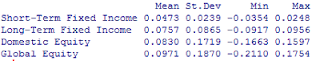Mean and Standard Deviation Annual Basis, Min and Max Monthly Basis

Otherwise, we can simply use our own intuition and judgment, perhaps informed by a number of the other methods.

In order to calculate the historical values of these asset classes I used 4 index funds from Vanguard covering the period from January, 1996 to January, 2013. These funds’ return performance is summarized in Figure C, which was calculated using the open-source statistical software R.
Figure D
Asset Class Data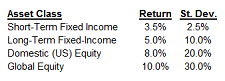In addition, estimates of expected future asset portfolio performance were reviewed such as those provided by JP Morgan.
Based on this activity, Figure D shows the expected annual rates of return and standard deviations that we will use in the simulation, and Figure E shows the R output of the correlation matrix between these asset classes. For purposes of this simulation we will assume that returns will vary according to the normal distribution.
Figure E
Asset Correlation Matrix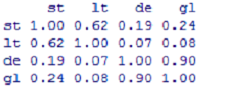st = Short-Term Fixed Income
lt = Long-Term Fixed Income
de = Domestic Equity
gl = Global Equity

Setting-Up the Simulation: Portfolio Decisions
As discussed in the last section, we want to target a point on the efficient frontier for our portfolio’s performance. There are equations that can do this, but the drawback to them is that they allow negative values in the target weights, which implies “shorting” those asset classes (to short a security you sell first, and buy back later, thus making money if the price falls while your trade is in effect). While shorting transactions occur and are perfectly legitimate, in my experience individual investors and conservative investment managers such as defined benefit trust funds do not undertake these even if they are possible.
Lacking the ability to use these equations, we can still set our targets using what amounts to “computational brute force” - calculate every possible combination of portfolio weight and then determine which of those lie on the frontier.
The left panel of Figure F shows the return and risk of the entire universe of portfolio combination using 5% intervals and our simulation assumptions. At this point we need to make a decision: what is going to be our target?
Figure F
Efficient Frontier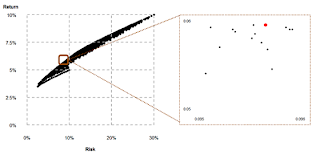By taking a “slice” of this universe close to one of our target values, we can find the portfolio combination that lies on the efficient frontier. This is shown in the right panel of Figure F. All portfolio combination lying within a range of 9.5% and 9.6% are shown, with the red dot signifying the one that delivers the highest return for that level of variation.
This efficient frontier portfolio is weighted as follows:
Short-Term Fixed Income       10%
Long-Term Fixed Income        60%
Domestic Equity                      20%
Global Equity                           10%

Using Figure A - Equation 1, we can

Figure G
Portfolio Variance Calculationcalculate that the expected return on this portfolio should be 5.95% (.1 * .035 + .6 * .05 + .2 * .08 + .1 * .1), and using Equation 2 its standard deviation to be 9.57%. Figure G shows the standard deviation calculation in Excel.

The final input is the investment amount. For purposes of this simulation, we will assume that the portfolio begins with ²100 (the symbol ²  stands for Treasury Café Monetary Units, freely convertible into any other currency at any exchange rate of your choosing), and that each month an additional ²100 is contributed, representing conditions one might expect to find in a trust funding situation.

Simulation Results
Each simulation performed produced a 360 month progression (30 years) of asset and portfolio values. The average return and standard deviation for each 30 year run was then stored. For each scenario – Rebalancing and No Rebalancing - this simulation was repeated 10,000 times. For the Rebalancing scenario, we executed transactions once per year to buy or sell in order to get back to the target percentages.
We targeted a

Figure H
Portfolio Standard Deviation Comparison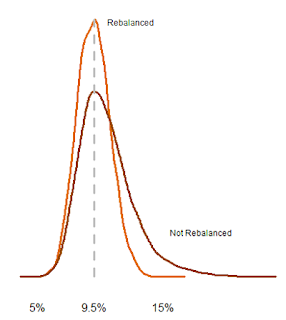standard deviation of about 9.5% as our target portfolio variance. Figure H shows the density curves (probabilities of each outcome) of each scenario’s 10,000 results. Clearly rebalancing keeps the portfolio a lot closer to the target standard deviation, as indicated by the fact that the rebalanced portfolio’s standard deviation density is “taller and skinnier” than the portfolio that was not rebalanced. This means that we are much more likely to attain our targets by rebalancing.

For the portfolios that were not rebalanced, the average standard deviation varies much more widely from our targets. For example, there were a significant number of outcomes where the portfolios that were not rebalanced would average standard deviations

Figure I
Portfolio Return Comparisonhigher than 15%, or over 50% from where we wanted it to be.

Figure I shows the average portfolio return over the 30 year time period for each scenario. As was the case in our look at standard deviation, rebalanced portfolios were closer to the target and did not vary as much as non-rebalanced ones. The portfolios that were not rebalanced had slightly higher returns, as you can notice by the gap between the two around the 10% mark.
Figure J

Figure J
Portfolio Ending Value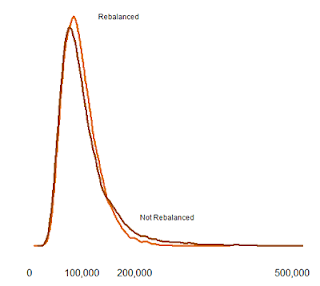Summary Table Values in 1,000's

shows the final values of the portfolios after 30 years. While the ending values were slightly higher for the non-rebalanced portfolios, the average “improvement” was only about
²8,000, less than 10% of the total values. This does not seem efficient given the up to 50% increases in standard deviation required to achieve them. Thus, while we might earn higher returns, it is almost a certainty that the non-rebalanced portfolio does not lie on the efficient frontier.

Key Takeaways
Investors aiming to achieve specific objectives with their investment portfolios need to rebalance their portfolios periodically in order to keep performance in line with targets.
Questions
·         How often do you rebalance your portfolio?
·         How would you improve the Monte Carlo methodology employed here?

Add to the discussion with your thoughts, comments, questions and feedback! Please share Treasury Café with others. Thank you!